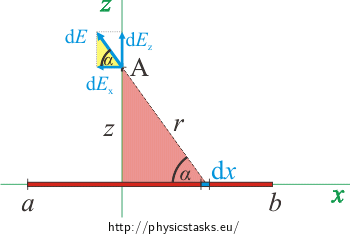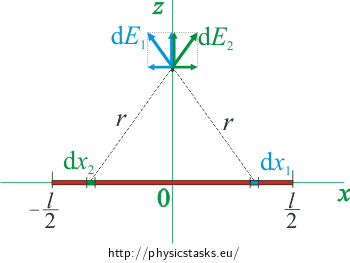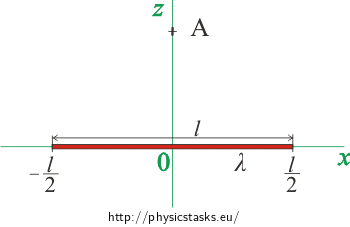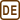## Charged line segment

Line segment with long L has linear density of charge λ. Calculate the electric field:

a) at height z anywhere above the line segment

b) at height z above the centre of the line segment

c) at height z above the line (infinitely long line segment)

Note:: Points b) and c) are special cases of point a)

• #### a) Hint: Picture and Comment

We choose a coordinate system at first and draw a picture.Note: We suppose in all tasks that the line segment (line) is charged with positive charge. The vector of electric field will be in opposite direction for negative charge.

• #### a) Hint: Connection with a point charge

Positive point charge Q creates electric field in distance r

$E\,=\,\frac{1}{4 \pi \varepsilon_0}\, \frac{Q}{r^2}\,.$

Direction of the vector of electric field is from the charge.

• #### a) Analysis

We choose a coordinate system at first. Put the line segment on axis x and point A on axis z in distance z from the origin of the coordinate system. In this case, the vector of electric field is in a plane xz .

We divide the line segment into small parts which will behave as point charges. We gain the total electric field as an integral of contributions of electric fields from constituent elements.

• #### a) Solution

We choose a coordinate system at first.

Put the line segment on axis x and point A on axis z in distance z from the origin of the coordinate system. Mark end points of the line segment a and b. Simultaneously, these are also x coordinates of end points of the line segment.Note: If we place the line segment as it is shown in the picture, then the coordinate on its left end point is minus, i.e. value a is minus.

We know, that point charge with value Q creates electric field in distance r:

$E\,=\, \frac{1}{4 \pi \varepsilon_0}\,\frac{Q}{r^2}\,.$

So, we divide the line segment into small parts with length dx which have charge dQ = λdx and which will behave as a point charge.Such a part of the line segment will in point A (which is in distance r) create electric field:

$\mathrm{d}E\,=\, \frac{1}{4 \pi \varepsilon_0}\,\frac{\mathrm{d}Q}{r^2}\,=\, \frac{1}{4 \pi \varepsilon_0}\,\frac{\lambda\mathrm{d}x}{r^2}\,.\tag{*}$

A vector of the electric field changes its direction for each individual contribution. So, we will calculate the value of the vector in its components. The component of the electric field in direction of y axis (i.e. perpendicularly to the picture) has for all contributions a zero value. We will use a yellow triangle in the next picture to formulate other components of the vector. We mustn´t forget that the component in the x axis is minus.$\mathrm{d}E_x\,=\,-\, \cos\alpha\,\mathrm{d}E$ $\mathrm{d}E_z\,=\, \sin\alpha\,\mathrm{d}E$

We use sides of the red triangle to formulate sine and cosine

$\cos\alpha\,=\, \frac{x}{r}$ $\sin\alpha\,=\, \frac{z}{r}$

and substitute it to previous equations.

$\mathrm{d}E_x\,=\, -\frac{x}{r}\,\mathrm{d}E$ $\mathrm{d}E_z\,=\,\frac{z}{r}\, \mathrm{d}E$

Now substitute from equation (*) for dE.

$\mathrm{d}E_x\,=\,- \,\frac{1}{4 \pi \epsilon_0}\,\frac{\lambda}{r^2}\,\frac{x}{r} \mathrm{d}x\,=\,- \,\frac{1}{4 \pi \epsilon_0}\,\frac{\lambda x}{r^ 3}\mathrm{d}x$ $\mathrm{d}E_z\,=\, \frac{1}{4 \pi \epsilon_0}\,\frac{\lambda}{r^2}\,\frac{z}{r}\mathrm{d}x\,=\, \frac{1}{4 \pi \epsilon_0}\,\frac{\lambda z}{r^3}\mathrm{d}x$

Formulate a distance r using the red triangle:

$r\,=\, \sqrt{x^2+z^2}$

and substitute it to the formula of both components.

$\mathrm{d}E_x\,=\,- \,\frac{1}{4 \pi \epsilon_0}\,\frac{\lambda x}{\left(x^2+z^2\right)^{\frac{3}{2}} }\mathrm{d}x$ $\mathrm{d}E_z\,=\, \frac{1}{4 \pi \epsilon_0}\,\frac{\lambda z}{\left(x^2+z^2\right)^{\frac{3}{2}} }\mathrm{d}x\tag{**}$

We gain components of the vector of electric field E as an integral of contributions of electric fields from constituent elements over the total length of the line segment.

$E_x\,=\,\int_a^b\mathrm{d}E_x$ $E_z\,=\,\int_a^b\mathrm{d}E_z$

Substitute from equation (**) for constituent elements and integrate it.

$E_x\,=\,- \,\int_a^b\frac{1}{4 \pi \epsilon_0}\,\frac{\lambda x}{\left(x^2+z^2\right)^{\frac{3}{2}} }\mathrm{d}x \,=\,- \,\frac{\lambda}{4 \pi \epsilon_0}\int_a^b\,\frac{x}{\left(x^2+z^2\right)^{\frac{3}{2}} }\mathrm{d}x$ $E_z\,=\,\int_a^b\frac{1}{4 \pi \epsilon_0}\,\frac{\lambda z}{\left(x^2+z^2\right)^{\frac{3}{2}} }\mathrm{d}x\,=\,\frac{\lambda z}{4 \pi \epsilon_0}\int_a^b\,\frac{1}{\left(x^2+z^2\right)^{\frac{3}{2}} }\mathrm{d}x$

A more detailed process of the calculation of integrals is in a separate section at the end of this task.

$E_x\,=\,- \,\frac{\lambda}{4 \pi \epsilon_0}\left[-\frac{1}{\sqrt{x^2+z^2} }\right]_a^b\,=\, \,\frac{\lambda}{4 \pi \epsilon_0}\left[\frac{1}{\sqrt{x^2+z^2} }\right]_a^b$ $E_z\,=\,\frac{\lambda z}{4 \pi \epsilon_0}\left[\frac{x}{z^2\sqrt{x^2+z^2} }\right]_a^b\,=\,\frac{\lambda z}{4 \pi \epsilon_0 z^2}\left[\frac{x}{\sqrt{x^2+z^2} }\right]_a^b$ $E_z\,=\,\frac{\lambda}{4 \pi \epsilon_0 z}\left[\frac{x}{\sqrt{x^2+z^2} }\right]_a^b$

Substitute end points of the line segment and gain a general formulation of components of the electric field.

$E_x\,=\,\frac{\lambda}{4 \pi \epsilon_0}\,\left(\frac{1}{\sqrt{b^2+z^2} }\,-\,\frac{1}{\sqrt{a^2+z^2}}\right)$ $E_y\,=\,0$ $E_z\,=\,\frac{\lambda}{4 \pi \epsilon_0 z}\left(\frac{b}{\sqrt{b^2+z^2} }\,-\,\frac{a}{\sqrt{a^2+z^2} }\right)$
• #### Hint to part b) and c)

You can use the results from part a) for calculation of the intensity above the centre of the line segment and the line, if you replace them by end points of line segment a and b.

If point A is placed above the centre of the line segment, we can use the length of the line segment to formulate end points a, b.

$$a\,=\,-\,\frac{l}{2} \hspace{20px}$$a $$\hspace{20px} b\,=\,\frac{l}{2}\,.$$

A line is actually a line segment, which has end points at infinity, so it is infinitely long, which means $$a \to -\infty$$, $$b \to \infty\,, \qquad$$ or $$\qquad l \to \infty$$ .

• #### b) and c) Analysis

Calculation of the intensity above the centre of the line segment is easier. A line segment is symmetrical with the centre in origin of the coordinate system. For every part of a line segment there is another part, which is symmetrical with axis z. Vectors of intensity from these parts of the line segment are symmetrical with axis z too (see the picture). In that case, the x components of these vectors have the same length and opposite direction so they subtract together. So, x and y components of the vector of the total intensity at point A is zero, which means that its orientation is in direction of axis z. We gain the magnitude of the total intensity by substituing into the result of the previous calculation.A line is a line segment, which has its end points at infinity. Because a line is symmetrical with axis z, vector of the total intensity has its orientation in the direction of axis z just as the line segment. We gain the magnitude of the vector if we enlarge the lenght of the line segment to infinity.

• #### b) Solution

For calculation of the intensity above the centre of the line segment we use the results from part a)

$E_x\,=\,\frac{\lambda}{4 \pi \epsilon_0}\,\left(\frac{1}{\sqrt{b^2+z^2}}\,-\,\frac{1}{\sqrt{a^2+z^2} }\right)$ $E_y\,=\,0$ $E_z\,=\,\frac{\lambda}{4 \pi \epsilon_0 z}\left(\frac{b}{\sqrt{b^2+z^2} }\,-\,\frac{a}{\sqrt{a^2+z^2} }\right)$

We can formulate end points by using the length of the line segment.

$a\,=\,-\frac{l}{2}$ $b\,=\,\frac{l}{2}$We calculate x component of intensity.

$E_x\,=\,\frac{\lambda}{4 \pi \epsilon_0}\,\left(\frac{1}{\sqrt{\frac{l^2}{4}+z^2} }\,-\,\frac{1}{\sqrt{\frac{l^2}{4}+z^2}}\right)\,=\,0$

Component is zero, which we deduce in part Analysis qualitatively only.Now we calculate z component of intensity which is the total magnitude of intensity too, because other two components are zero.

$E_z\,=\,\frac{\lambda}{4 \pi \epsilon_0 z}\left( \frac{\frac{l}{2}}{\sqrt{\frac{l^2}{2^2}+z^2} }\,-\,\frac{-\frac{l}{2}}{\sqrt{\frac{l^2}{2^2}+z^2} }\right)$

We add expressions in brackets and modify the fraction below the root.

$E_z\,=\,\frac{\lambda}{4 \pi \epsilon_0 z} \,\frac{l}{\sqrt{\frac{l^2}{4}+z^2} }\,=\,\frac{\lambda}{4 \pi \epsilon_0 z} \,\frac{l}{\sqrt{\frac{l^2+4z^2}{4}} }\,=\,\frac{\lambda}{4 \pi \epsilon_0 z} \,\frac{l}{\frac{\sqrt{l^2+4z^2}}{2} }$ $E_z\,=\,\frac{\lambda}{2 \pi \epsilon_0 z} \,\frac{l}{\sqrt{l^2+4z^2} }$

So we gain magnitude of the total intensity of the electric field above the centre of the charged line segment.

$E_x\,=\,0$ $E_y\,=\,0$ $E_z\,=\,\frac{\lambda}{2 \pi \epsilon_0 z} \,\frac{l}{\sqrt{l^2+4z^2} }$

The vector of intensity has its orientation in direction of axis z and it has a magnitude

$E\,=\,\frac{\lambda}{2 \pi \epsilon_0 z} \,\frac{l}{\sqrt{l^2+4z^2} }\,.$
• #### c) Solution

For expressing a formula for the intensity above the line we use the result from part b). On the basis of that we know that the vector of the electric intensity above the centre of the charged line segment with length l has its orientation in direction of axis z and its magnitude is

$E\,=\,\frac{\lambda}{2 \pi \epsilon_0 z} \,\frac{l}{\sqrt{l^2+4z^2} }\,.$

Because a line is an infinite line segment we must calculate the value of intensity E for l which approaches infinity.

$E\,=\, \lim_{\small l \tiny \to \infty } \,\frac{\lambda}{2 \pi \epsilon_0 z} \,\frac{l}{\sqrt{l^2+4z^2} }\,=\,\frac{\lambda}{2 \pi \epsilon_0 z} \, \lim_{\small l \tiny \to \infty } \,\frac{l}{\sqrt{l^2+4z^2} }$

Length l is much bigger than distance z, i.e. l >> z. So we can ignore term z2 in denominator of fraction towards l2.

$\lim_{\small l \tiny \to \infty } \,\large\frac{l}{\sqrt{l^2+4z^2}}\,=\,\lim_{ \small l \tiny \to -\infty }\large \,\frac{l}{\sqrt{l^2}}$

Calculate the root in denominator of fraction.

$\lim_{ \small l \tiny \to \infty }\, \large \frac{l}{\sqrt{l^2}}\,=\,\lim_{ \small l \tiny \to \infty } \large\,\frac{l}{|l|}\,=\,\lim_{ \small l \tiny \to \infty}\large\, \frac{l}{l}\,=\,\lim_{ \small l \tiny \to \infty }\large \, 1\,=\,1$

We substitute the result of the limit into the formula for magnitude of intensity E and we gain that the charged line creates in distance z electric field with intensity:

$E\,=\,\frac{\lambda}{2 \pi \epsilon_0}\,\frac{1}{ z}\,.$

Comment: We can calculate intensity from the result from part a). The method is very similar but more lengthy.

a) If a line segment is located on x between points with coordinates [a; 0; 0] and [b; 0; 0] and point A, in which we find out the intensity, has coordinates [0; 0; z], then the vector of intensity E has components:

$E_x\,=\,\frac{\lambda}{4 \pi \epsilon_0}\,\left(\frac{1}{\sqrt{b^2+z^2} }\,-\,\frac{1}{\sqrt{a^2+z^2} }\right)$ $E_y\,=\,0$ $E_z\,=\,\frac{\lambda}{4 \pi \epsilon_0 z}\left(\frac{b}{\sqrt{b^2+z^2} }\,-\,\frac{a}{\sqrt{a^2+z^2} }\right)$

b) In distance z above the centre of the line segment the vector of intensity has its direction perpendicularly to the line segment and has a magnitude

$E\,=\,\frac{\lambda}{2 \pi \epsilon_0 z} \,\frac{l}{\sqrt{l^2+4z^2} }\,.$

c) A charged line creates in distance z an electric field with intensity:

$E\,=\,\frac{\lambda}{2 \pi \epsilon_0}\,\frac{1}{ z}\,.$

The vector of intensity has its direction perpendicularly to the line.

• #### A detailed calculation of integrals from part a)

In this section we calculate integrals from section a) Solution.

The first integral  – calculation of component Ex:

$E_x\,=\,-\,\frac{1}{4 \pi \varepsilon_0} \int_a^b \frac{x}{\left( x^2\,+\,z^2\right)^{\frac{3}{2}}}\,\mathrm{d}x$

At first we calculate the integral as indefinite. We will calculate this integral using substitution:

$t\,=\,x^2\,+\,z^2$ $\mathrm{d}t\,=\,2x\,\mathrm{d}x \hspace{20px} \Rightarrow \hspace{20px} x\,\mathrm{d}x\,=\,\frac{\mathrm{d}t}{2}$

Substitute it into integral.

$\int \frac{x}{\left( x^2\,+\,z^2\right)^{\frac{3}{2}}}\,\mathrm{d}x\,=\,\int \frac{\mathrm{d}t}{2t^{\frac{3}{2}}} \,=\,\frac{1}{2}\int t^{-\frac{3}{2}}\,\mathrm{d}t\,=\,-\,\frac{1}{2} \,\frac{t^{-\frac{1}{2}}}{\frac{1}{2}}$

Reduce 1/2 and substitute it instead of t.

$\int \frac{x}{\left( x^2\,+\,z^2\right)^{\frac{3}{2}}}\,\mathrm{d}x\,=\, - \frac{1}{\sqrt{t}}$ $\int \frac{x}{\left( x^2\,+\,z^2\right)^{\frac{3}{2}}}\,\mathrm{d}x\,=\, - \frac{1}{\sqrt{x^2\,+\,z^2}}$

When we substitute inside limits, we gain the result

$E_x\,=\,-\,\frac{1}{4 \pi \varepsilon_0} \left[ - \frac{1}{\sqrt{x^2\,+\,z^2}} \right]_a^b$

The second integral – calculation of component Ez:

The second integral

$E_z\,=\,\frac{\lambda z}{4 \pi \epsilon_0}\int_a^b\,\frac{1}{\left(x^2+z^2\right)^{\frac{3}{2}} }\mathrm{d}x$

At first we calculate the second integral again as indefinite and use the substitution too, but this time the calculation is difficult. A suitable substitution is

$x\,=\,z \,\sinh \,t$ $\mathrm{d}x\,=\,z\,\cosh\, t \,\mathrm{d}t$

Substitute it to integral and edit the integral before the calculation.

$\int\,\frac{1}{\left(x^2+z^2\right)^{\frac{3}{2}} }\mathrm{d}x\,=\, \int\frac{z\,\cosh \,t \,\mathrm{d}t}{\left(z^2\,\sinh^2t+z^2\right)^{\frac{3}{2}}} \,=\, \int\frac{z\,\cosh \, t \,\mathrm{d}t}{\left(z^2\right)^{\frac{3}{2}} \left(\sinh^2t+1\right)^{\frac{3}{2}}} \,=\,$

Reduce z and use formula cosh2t = sinh2t + 1

$\,=\, \int\frac{z\,\cosh t \,\mathrm{d}t}{z^3 \left(\cosh^2t\right)^{\frac{3}{2}} }\,=\, \int\frac{\cosh t \,\mathrm{d}t}{z^2 \,\cosh^3t }\,=$

Now we reduce the expression, remove constants before the integral and then we calculate the integral.

$=\, \frac{1}{z^2}\int\frac{\mathrm{d}t}{ \,\cosh^2 t }\,=\, \frac{1}{z^2} \,\tanh\, t\,=\, \frac{1}{z^2}\, \frac{\sinh\, t}{\cosh\, t} \,=\, \frac{1}{z^2}\frac{\sinh \,t}{\sqrt{1+\sinh^2 t}} \,=$

Put x back from the substitution and edit the complex fraction.

$\,=\, \frac{1}{z^2} \frac{\frac{x}{z}}{\sqrt{1+\frac{x^2}{z^2}}} \,=\, \frac{1}{z^2} \frac{x}{z \sqrt{\frac{x^2+z^2}{z^2}}} \,=\, \frac{1}{z^2}\, \frac{x}{ \sqrt{x^2+z^2}}$

Now we can substitute in limits.

$E_z\,=\, \frac{\lambda z}{4 \pi \epsilon_0}\frac{1}{z^2}\, \left[ \frac{x}{ \sqrt{x^2+z^2}} \right]_a^b \,=\, \frac{\lambda}{4 \pi \epsilon_0\,z}\,\left[ \frac{x}{ \sqrt{x^2+z^2}} \right]_a^b$
• #### Link: How to calculate the intensity in the surroundings of the line differently?

We can calculate the intensity of the electric field in the surroundings of the line using Gauss´ law. This method is publish in task Pole nabité přímky (only in Czech).